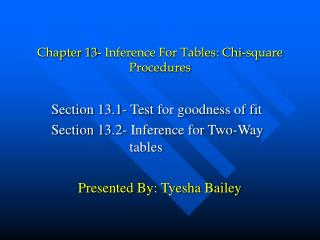DownloadDownload PresentationChapter 13- Inference For Tables: Chi-square Procedures

# Chapter 13- Inference For Tables: Chi-square Procedures

Télécharger la présentation## Chapter 13- Inference For Tables: Chi-square Procedures

- - - - - - - - - - - - - - - - - - - - - - - - - - - E N D - - - - - - - - - - - - - - - - - - - - - - - - - - -
##### Presentation Transcript

1. Chapter 13- Inference For Tables: Chi-square Procedures Section 13.1- Test for goodness of fit Section 13.2- Inference for Two-Way tables Presented By: Tyesha Bailey

2. Introduction: Things to learn throughout the chapter • Goodness of Fit • Calculate expected values for every category in a distribution, chi-squared statistic, and P-value • State the null and alternative hypothesis for differences between two distributions • Use components of the chi-square statistic to identify the most important deviations between observed and expected counts • Two-Way Tables • Arrange data for successes and failures into a two-way table

3. Introduction: Things to learn throughout the chapter Continued…. • Use percents to describe relationships between two categorical variables • Interpreting Chi-square tests of Independence • Identify expected cell counts, chi-square statistic, and its P-value using the calculator • Explain what the null hypothesis the chi-square statistic tests in a specific two-way table • Determine the deviations from the null hypothesis by using percents, comparison of expected and observed counts, and the components of chi-square statistic

4. Introduction: Things to learn throughout the chapter Continued…. • Doing Chi-square Tests • Calculate the expected value of any observed count in a two-way table • Calculate the component of the chi-square statistic for any cell, • Give the degrees of freedom for the chi-square statistic • Use chi-square critical values to approximate the P-value of a chi-square test

5. Vocabulary: Words to be familiar with throughout the chapter • Chi-square test for goodness of fit- test that shows the difference between the observed sample and the hypothesis population distributions • Chi-square distributions- Family of distributions that take only positive values and are skewed right • Degrees of freedom- chi-square distribution specified by one parameter • Goodness of fit test- helps determine whether a population has a certain hypothesized distribution, and expressed as percents of population

6. Vocabulary: Words to be familiar with throughout the chapter • Expected counts- any cell of a two-way table when the alternative hypothesis is true • Chi-square statistic- a measure of how far the observed counts in a two-way table are from the expected counts.

7. Examples Of Topics/ Equations • Two-way table • Expected Count • Chi-square, Degrees of freedom, P-value

8. Chi-square test for goodness of fit Chi-square test for independence Goodness of fit test Degrees of freedom Two-way tables P-values Expected Counts Chi-square Statistic All equations in the chapter Conclusion of Chapter 13/ Need to know Topics